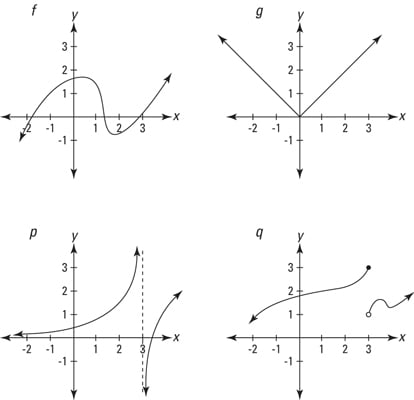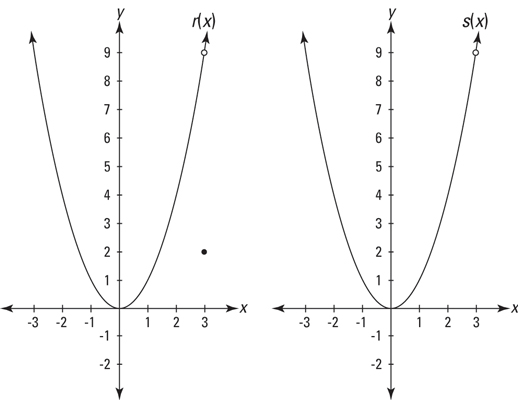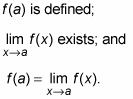##### Linear Algebra For DummiesHere you’ll learn about continuity for a bit, then go on to the connection between continuity and limits, and finally move on to the formal definition of continuity.

## Common sense definition of continuity

Continuity is such a simple concept — really. A continuous function is simply a function with no gaps — a function that you can draw without taking your pencil off the paper. Consider the four functions in this figure.Whether or not a function is continuous is almost always obvious. The first two functions in this figure — f (x) and g(x) — have no gaps, so they’re continuous. The next two — p(x) and q(x) — have gaps at x = 3, so they’re not continuous.

That’s all there is to it! Well, not quite. The two functions with gaps are not continuous everywhere, but because you can draw sections of them without taking your pencil off the paper, you can say that parts of those functions are continuous.

And sometimes, a function is continuous everywhere it’s defined. Such a function is described as being continuous over its entire domain, which means that its gap or gaps occur at x-values where the function is undefined. The function p(x) is continuous over its entire domain; q(x), on the other hand, is not continuous over its entire domain because it’s not continuous at x = 3, which is in the function’s domain. Often, the important issue is whether a function is continuous at a particular x-value. It is unless there is a gap there.

All polynomial functions are continuous everywhere. All rational functions — a rational function is the quotient of two polynomial functions — are continuous over their entire domains.

## The continuity-limit connection

With one big exception (which you’ll get to in a minute), continuity and limits go hand in hand. For example, consider again functions f, g, p, and q. Functions f and g are continuous at x = 3, and they both have limits at x = 3. Functions p and q, on the other hand, are not continuous at x = 3, and they do not have limits at x = 3. The exception to the rule concerns functions with holes. Actually, when you come right down to it, the exception is more important than the rule. Consider the two functions in the next figure.These functions have gaps at x = 3 and are obviously not continuous there, but they do have limits as x approaches 3. In each case, the limit equals the height of the hole. An infinitesimal hole in a function is the only place a function can have a limit where it is not continuous.

Both functions in the figure have the same limit as x approaches 3; the limit is 9, and the facts that r(3) = 2 and that s(3) is undefined are irrelevant. For both functions, as x zeros in on 3 from either side, the height of the function zeros in on the height of the hole — that’s the limit.

The limit at a hole is the height of a hole.

## Formal definition of continuity

A function f (x) is continuous at a point x = a if the following three conditions are satisfied:Just like with the formal definition of a limit, the definition of continuity is always presented as a 3-part test, but condition 3 is the only one you need to worry about because 1 and 2 are built into 3. You must remember, however, that condition 3 is not satisfied when the left and right sides of the equation are both undefined or nonexistent.